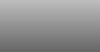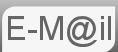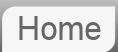# Clue to Puzzle #2: 90 coins and an old-fashioned balance

2. You are given a set of scales and 90 coins. The scales are of the same type as above. You must pay \$100 every time you use the scales.

The 90 coins appear to be identical. In fact, 89 of them are identical, and one is of a different weight. Your task is to identify the unusual coin and to discard it while minimizing the maximum possible cost of weighing (another task might be to minimizing the expected cost of weighing). What is your algorithm to complete this task? What is the most it can cost to identify the unusual coin?

I've seen several answers to this question, 4 at least and they are mostly very hard. Like the answer to Question 1 but much longer as we make the transition to 90 coins. The best answer I have seen and the one that we cover in the answer is to actually use the answer from Question 1 to begin to tackle the 90 coin version.

I can hint a little further if you wish...

## Number Theory and the Number of Weighings

At the end of the first answer we started to look at what number theory could tell us about the 12 marble/coin problem '...each individual weighing has 3 possible outcomes, (left, right & balanced,) and there being 3 weighings there are 33=27 possible results in total. Reflections are not useful to us so there is enough information from 3 independent weighings to determine the results for 13.5 marbles....' It is not difficult to see how we can extend this to determine how many weighings are required here. If it's not completely clear the first three is because of the three possible outcomes of each weighing, and the power of three is because of the three individual weighings.

We now have the tools to begin to look at how many coins we can solve for any given number of weighings
2: 32/2 = 4.5
3: 33/2 = 13.5
4: 34/2 = 40.5
5: 35/2 = 121.5
6: 36/2 = 364.5

This suggests there should be enough information obtained from from 5 weighings such that we should be able to identify the moody coin within that number of weighings. Certainly it proves beyond doubt that 4 weighings is not enough. Thus the answer to the first and easiest part of the question is likely to be in 5 weighings or \$500.

Where next?

© Nigel Coldwell 2004 -  – The questions on this site may be reproduced without further permission, I do not claim copyright over them. The answers are mine and may not be reproduced without my expressed prior consent. Please inquire using the link at the top of the page. Secure version of this page.

PayPal
I always think it's arrogant to add a donate button, but it has been requested. If I help you get a job though, you could buy me a pint! - nigel